## Introduction

A fundamental challenge in studying complex networked systems is to reveal the interplay between network structure and function1,2. Here we tackle this challenge by investigating a classical notion in graph theory, that is, articulation points. A node in a network is an articulation point (AP) if its removal disconnects the network or increases the number of connected components in the network3,4 (Fig. 1a). Those APs can be easily identified using a linear-time algorithm based on depth-first search5. It has been found that APs play important roles in ensuring the robustness and connectivity of many real-world networks. For example, in infrastructure networks such as air traffic networks or power grids, APs, if disrupted or attacked, pose serious risks to the infrastructure6,7. In wireless sensor networks, failures of APs will block data transmission from one network component to others8. In the yeast protein–protein interaction network, lethal mutations are enriched in the group of highly connected proteins that are APs9. Analysis of APs hence provides us a different angle to systematically investigate the structure and function of real-world networks.Figure 1: Articulation points and the greedy articulation points removal process.

Despite the importance of APs in ensuring the robustness and connectivity of many real-world networks, we still lack a deep understanding on the roles of APs in many complex networks. Can we design an AP-based attack strategy to more efficiently destroy malicious networks? Can we develop an AP-based network decomposition method to better reveal the organizing principles of complex networks? What happens if we keep removing APs from a random graph or a real network? Will there be a core left? If yes, what’s the implication of such a core in terms of structural integrity and functionality of the network? How to quantify if a real network has overrepresented or underrepresented APs comparing to its randomized counterparts? In this article we offer an analytical framework to study those fundamental issues pertinent to APs in both real networks and random graphs, harvesting a series of interesting results.

## Results

### Articulation point–targeted attack

Representing natural vulnerabilities of a network, APs are potential targets of attack if one aims for immediate damage to a network. Note that the removal of an AP in a network may lead to the emergence of new APs in the remainder of the network, that is, new potential targets of attack (Fig. 1b). This fact inspires us to design a brute-force AP-targeted attack (APTA) strategy: iteratively remove the most destructive AP that will cause the most nodes disconnected from the giant connected component (GCC) of the current network. Given a limited ‘budget’ (that is, the number of nodes to be removed), this APTA strategy is very efficient in reducing the GCC, compared with strategies based on other node centrality measures, such as degree10,11 and collective influence12. Indeed, we find that for a small fraction of removed nodes APTA leads to the fastest reduction of GCC for a wide range of real-world networks from technological to infrastructure, biological, communication, and social networks (Supplementary Note 1; Supplementary Fig. 1). Depending on the initial network structure, APTA would either completely decompose the network or result in a residual GCC that occupies a finite fraction of the network. This residual GCC is a biconnected component (or bicomponent), in which any two nodes are connected by at least two independent paths and hence no AP exists5. For accuracy, we will call it residual giant bicomponent (RGB) hereafter. This RGB naturally represents a core that maintains the structural integrity of the network.

### Greedy articulation points removal

We also find that the identification and removal of APs provide us a new perspective on the organizational principles of complex networks. For example, in the terrorist communication network of the 9/11 attacks on U.S. (Fig. 1a), each AP member (shown in red) can be considered as a messenger of a particular subnetwork, because any information exchange between that subnetwork and the rest of the network passes through the AP13. All the APs and their associated subnetworks in the original network constitute the first layer of the terrorist network. After removing all the APs in the original network, the first layer is peeled off, new APs emerge and the second layer of the network is exposed. We can repeat this greedy APs removal (GAPR) process until there is no AP left in the network. Note that at each step we simultaneously remove all the APs present in the current network. Figure 1 illustrates this network decomposition process in the 9/11 terrorist communication network, which has 62 terrorists. We find that this network consists of three layers and an RGB of 26 nodes. (Note that the RGB associated with the GAPR process is similar to but not necessarily the same as that of the APTA process, see Supplementary Note 2; Supplementary Fig. 2.) Interestingly, among those 26 RGB nodes, 16 of them are hijackers in the 9/11 terrorist attack, which in total has 19 hijackers. In a sense, this RGB serves as a core maintaining the functionality of this covert network, which has a particular goal—hijacking. Note that some of the hijackers in the RGB are not hubs (that is, highly connected nodes), but only have two or three neighbours in the network. Hence they cannot be easily identified through traditional network decomposition methods, for example, maximum clique3,4, k-core decomposition14,15 and t-core decomposition16,17,18, which are designed to uncover or extract a dense core structure consisting of highly connected nodes (Supplementary Note 3; Supplementary Fig. 3).

Interestingly, we find that the two RGBs associated with the APTA and GAPR processes significantly overlap for many real-world networks (Supplementary Note 2; Supplementary Fig. 2). Note that, compared with APTA, the GAPR process is deterministic and avoids the optimization of the damage caused by nodes removal, which make it analytically solvable. Hereafter we focus on the RGB obtained from the GAPR process.

### Articulation points and residual giant bicomponent in real networks

The results presented in the previous subsections prompt us to study the fraction of APs (nAP:=NAP/N) and the relative size of the RGB (nRGB:=NRGB/N) in a wide range of real-world networks. Here NAP, NRGB and N represent the number of APs, the number of nodes in the RGB (obtained from the GAPR process), and the number of nodes in the whole network, respectively.

We find that many real networks have a non-ignorable fraction of APs and a rather small RGB (Fig. 2a). One may expect that infrastructure networks should have a relatively small fraction of APs and a large RGB, and hence are very robust against AP removal. Interestingly, this is not the case. The power grids in two regions of U.S. have almost the largest fraction (24%) of APs among all the real networks analysed in this work. And they have almost no RGB. The road networks of three states in U.S. have almost 20% of APs, and a small RGB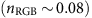. These results suggest that infrastructure networks are apparently not optimized with respect to AP removal. Indeed, because of the high cost of adding new links (for example, connecting two power stations with high-voltage transition lines, or connecting two cities with a new highway), infrastructure networks typically lack a high redundancy, but are often optimized with respect to other criteria, such as social profitability. By contrast, among all the 28 food webs we analysed, 22 of them have no APs (and hence nRGB=1). In other words, those ecological networks tend to be biconnected and the extinction of one species will not disconnect the whole community. This high structural robustness could be because of evolutionary inter-species interactions across the whole community19.Figure 2: Articulation points and the residual giant bicomponent in real networks.

More interestingly, we find that most of the real networks analysed here have either a very small RGB or a rather big one (see Fig. 2a, light magenta and turquoise regions). Later we will show that this phenomenon is related to a discontinuous phase transition associated with the GAPR process.

To identify the topological characteristics that determine these two quantities (nAP and nRGB), we compare nAP (or nRGB) of a given real network with that of its randomized counterpart. To this aim, we randomize each real network using a complete randomization procedure that turns the network into an Erdős-Rényi (ER) type of random network with the number of nodes N and links L unchanged20. We find that most of the completely randomized networks possess very different nAP (or nRGB), comparing to their corresponding real networks (Fig. 2b,c). This indicates that complete randomization eliminates the topological characteristics that determine nAP and nRGB. By contrast, when we apply a degree-preserving randomization, which rewires the links among nodes, while keeping the degree k of each node unchanged, this procedure does not alter nAP and nRGB significantly (Fig. 2d,e). In other words, the characteristics of a network in terms of nAP and nRGB is largely encoded in its degree distribution P(k). Most of the real-world networks display slightly smaller nAP and bigger nRGB than their degree-preserving randomized counterparts. We attribute these differences to higher-order structure correlations, such as clustering21 and degree assortativity22, which are eliminated in the degree-preserving randomization.

### Analytical framework of the greedy articulation points removal process

The results of nAP and nRGB in real-world networks encourage us to analytically calculate nAP and nRGB for networks with prescribed degree distributions23. To achieve that, we analyse the GAPR process on infinitely large networks and explore in depth the effect of different degree distributions on nAP and nRGB. Consider the discrete-time dynamics of the deterministic GAPR process, which generates a series of snapshots for the remainder network with a clear temporal order {0, 1,..., t,...., T}. Here, T is the total number of GAPR steps, which is also the number of layers peeled off during the GAPR process. We denote the fraction of APs and the relative size of the GCC in the original network as nAP(0) and nGCC(0), respectively. Removal of the original APs leads to a new fraction of APs nAP(1) and a smaller GCC of relative size nGCC(1). We repeat this process and denote the fraction of APs and the relative size of the GCC of the network snapshot at time step t as nAP(t) and nGCC(t), respectively. At the end of the GAPR process, we have nAP(T)=0 and nGCC(T)=nRGB. On the basis of the configuration model of uncorrelated random networks23,24,25, we can analytically calculate nAP(t) and nGCC(t) for networks with arbitrary degree distributions at any time step t. This enables us to further compute T and nRGB. See Methods section and Supplementary Note 4 for the details of our analytical framework of the GAPR process.

### Articulation points in classical model networks

The analytical framework of the GAPR process enables us to calculate various quantities of interests. We first investigate the fraction of APs in the original network, that is, nAP=nAP(0). We calculate nAP in two canonical model networks: (1) ER random networks with Poisson degree distributions P(k)=ecck/k!, where c is the mean degree (hereafter we also use c to denote the mean degree of a general network); and (2) scale-free (SF) networks with power-law degree distributions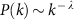, where λ is often called the degree exponent (Fig. 3a,b). The fraction of APs is trivially zero in the two limits c→0 and c→∞, and reaches its maximum at a particular mean degree cAP. For ER networks, we find that cAP=1.41868, which is larger than cp=1, the critical point of ordinary percolation where the GCC emerges1,2.Figure 3: Fraction of articulation points in two canonical model networks.

The phenomena that cAP>cp is even more prominent for SF networks generated by the static model26, where cp(λ)<1 and cAP(λ)>1.41868. This is because, for those SF networks, even though the GCC emerges at lower cp(λ), its relative size is rather small at the initial stage of its emergence and the network is more fragmented in FCCs, which results in larger cAP(λ) (Fig. 3b) (see Supplementary Note 5 for details).

### Percolation transitions associated with greedy articulation points removal

We now systematically study the behaviours of nGCC(t) and nRGB, as functions of the mean degree c for infinitely large ER networks. To emphasize the c-dependence, hereafter we denote nGCC(t) and nRGB of ER networks as nGCC(t, c) and nRGB(c), respectively. To systematically characterize the percolation transitions, various quantities will be analysed, such as the critical mean degree, critical exponents, the jump size of the order parameter at criticality, and so on.

As shown in Fig. 4a (grey lines), after any finite steps of GAPR, the GCC always emerges in a continuous manner, suggesting a continuous phase transition. Hereafter we will call it GCC percolation transition. For t steps of GAPR, the GCC percolation transition displays a critical phenomenon: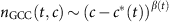for (cc*(t))→0+, where c*(t) is the critical mean degree, that is, the percolation threshold, and β(t) is the critical exponent. We find that as t increases, c*(t) becomes larger and larger, but eventually converges to c*(∞)=c*=3.39807. Note that, for any finite t, the critical exponent β(t) associated with the GCC percolation transition is the same: β(t)=βGCC=1 (see Fig. 4c, grey lines).

By contrast, if we allow for infinite steps of GAPR (that is, we stop the process only if there is no AP left), the size of the resulting GCC (that is, the RGB), denoted as nRGB(c), displays a remarkable discontinuous phase transition: nRGB(c) abruptly jumps from zero (when c<c*) to a finite value at c*, and then increases with increasing c (see Fig. 4a black line). Hereafter we will call it RGB percolation transition. If we denote the jump size as Δ, we find that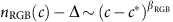with critical exponent βRGB=1/2 when (cc*)→0+ (Fig. 4c, black line), suggesting that the RGB percolation transition is actually a hybrid phase transition14,27,28. In other words, nRGB(c) has a jump at the critical point c* as a first-order phase transition but also has a critical singularity as a second-order phase transition. Interestingly, the GCC and RGB percolation transitions have completely different critical exponents associated with their critical singularities (Fig. 4c).

We also calculate the total number of GAPR steps T(c) needed to remove all APs of an infinitely large ER network of mean degree c (Fig. 4b). We find that T(c) is finite for c<c*; diverges when (cc*)→0; and is infinite for any c>c*. The divergence of T(c) displays a scaling behaviour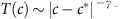with critical exponent γ=1/2 when (cc*)→0 (Fig. 4d, magenta line).

The nature of the discontinuous RGB percolation transition and the behaviour of T(c) can be revealed by analysing the dynamics of the GAPR process. In particular, we can calculate nGCC(t, c), nAP(t, c), as well as a key quantity in the GAPR process, that is, the average number of newly induced APs per single AP removal: η(t, c)=nAP(t, c)/nAP(t−1, c) for t>0 and at different mean degrees c (Fig. 4e–g).

For c>c* (supercritical region), the fraction of APs exponentially decays as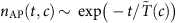after an initial transient time (Fig. 4f, turquoise lines), where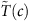is the characteristic time scale. In this region, with increasing t, η(t, c) quickly reaches an equilibrium value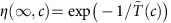, which is smaller than 1 (Fig. 4g, turquoise lines). Consequently, nGCC(t, c) converges to a finite value for t→∞ (Fig. 4e, turquoise lines), resulting in a finite nRGB. Since T(c) is infinite in this region, we can useto characterize the relaxation behaviour of GAPR process. We find thatincreases as c decreases (Fig. 4b, turquoise line), and diverges as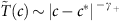*|γ+ with critical exponent γ+=1/2 when (cc*)→0+ (Fig. 4d, turquoise line). Note that as c decreases and approaches c* from above, the equilibrium value η(∞, c) gradually approaches 1 (Fig. 4g).

When (cc*)→0+ (that is, right above the criticality), the fraction of APs decays in a power-law manner for large t, that is,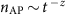with z=2 (Fig. 4f and inset, black line), rendering η(∞, c)=1 (Fig. 4g, black line). Consequently, nGCC(t, c) converges to a finite value in the t→∞ limit (Fig. 4e, black lines), leading to a finite nRGB. The fact η(∞, c)=1 suggests that in average every removed AP will induce one new AP at the next time step, and hence the GAPR process will continue forever. This explains why T(c*) diverges. Note that the equilibrium value η(∞, c)=1 can only be reached when nAP(t, c) displays a power-law decay as t→∞. This is because, as long as nAP(t, c) is finite at any finite t, the GAPR will gradually dilute the network, rendering a larger and larger value of η(t, c) as t grows.

When (cc*)→0 (that is, right below the criticality), as well as in the entire subcritical region (c<c*), after an initial decay, nAP(t, c) begins to exponentially grow with increasing t (Fig. 4f, magenta lines). Consequently, η(t, c) is initially <1, but then becomes drastically larger than 1 (Fig. 4g, magenta lines), which causes nGCC(t, c) quickly decays to zero (Fig. 4e, magenta lines), and hence T(c) is finite and the RGB dose not exist. The sudden collapse of the RGB upon an infinitesimal decrease in c suggests the discontinuous nature of the RGB percolation transition in ER networks. Note that at time step T, the network will break into pieces and there is no AP left. Hence in the last few GAPR steps the growth of nAP(t, c) will slow down and eventually decrease (Fig. 4f,g, tails in the magenta lines).

For finite-sized networks sampled from a network ensemble with a prescribed degree distribution, the value of nRGB at criticality c* is subject to large sample-to-sample fluctuations, being either zero or a large finite value (Fig. 5a and inset), which is another evidence of discontinuous phase transition29. This discontinuous phase transition also partially explains the fact that real-world networks have either a very small or a rather big RGB (Fig. 2a).Figure 5: Residual giant bicomponent percolation transition and the phase diagram.

The nature of the RGB percolation transition in SF networks is qualitatively the same as that in ER networks. The transition from the non-RGB phase to the RGB phase is discontinuous (Fig. 5a). The critical point c*(λ) increases with decreasing λ. Also, the jump of the RGB relative size at criticality increases as λ approaches 2 (Fig. 5a).

The cλ phase diagram of SF networks is shown in Fig. 5b. The whole diagram consists of three phases. For c<cp(λ) (grey region), there exists no GCC in the network, and hence no RGB. For cp(λ)<c<c*(λ) (light blue region), even though the GCC may survive after certain finite steps of GAPR, the RGB still does not exist. Since in both regimes there is no RGB, we call them non-RGB-I phase and non-RGB-II phase, respectively. The transition between these two phases is the ordinary GCC percolation transition, which is continuous (thick dashed line). Note that, in non-RGB-II phase, the phase transition associated with the emergence the GCC after any finite t steps of GAPR is still continuous (thin dot-dashed lines). For c>c*(λ) (light yellow region), the network suddenly has an RGB. This regime is referred to as the RGB phase. As we mentioned above, the transition between the non-RGB-II phase and the RGB phase is discontinuous (thick solid line). We have performed extensive numerical simulations to confirm our analytical results (Supplementary Note 6; Supplementary Figs 4–6).

Structural transitions in complex networks have been extensively studied and found to affect many network properties10,11,12,14,29,30,31,32,33,34,35,36,37,38,39,40,41,42,43. Here we show for the first time that there exists two different types of percolation transitions associated with the removal of APs.

## Discussion

In this article, we systematically investigate AP-related issues in complex networks. Many interesting phenomena of APs are discovered and explained for the first time. On the empirical side, we proposed two AP-based applications: a network attack strategy (APTA) and a network decomposition method (GAPR). We found that, given a limited ‘budget’ (that is, the number of nodes to be removed), our APTA strategy is more efficient in reducing the GCC of the network than other existing strategies. In revealing the core-periphery structure of complex networks, our GAPR method is quite different from traditional network decomposition methods in the sense that our identified core may include low-degree nodes. Those sparsely connected nodes can be functionally very important, but they are always ignored in traditional decomposition methods. On the theoretical side, we proposed an analytical framework to calculate various AP-related properties, among which the emergence of the RGB as a discontinuous percolation transition is of great theoretical interest. This finding also provides a theoretical explanation of the empirical findings that most of the real-world networks have either a very small RGB or a rather big one.

Taken together, our results offer a different perspective on the organizational principles of complex networks, shed light on the design of more resilient infrastructure networks and more effective destructions of malicious networks, and open new avenues to deepening our understanding of complex networked systems. Since the identification of APs also helps us better solve other challenging problems, for example, the calculation of determinants of large matrices44, and the minimum vertex cover problem on large graphs (a classical NP-hard problem)45, we anticipate that our results on APs will trigger more research activities on those problems as well.

## Methods

### Theoretical analysis of greedy articulation points removal process

Our theoretical treatment of the GAPR process is based on the local tree approximation, which assumes in the thermodynamics limit (that is, network size N→∞) there are no finite loops in a network and only infinite loops exist23,24,25,28. This approximation allows us to use the convenient techniques of random branching processes to solve the GAPR process on large uncorrelated random networks (Supplementary Note 4). Note that the local tree approximation is only exact for networks with finite second moment of the degree distribution. However, it has been demonstrated in various network problems that this approximation can obtain very accurate results even for networks with diverging second moment of the degree distribution28. Here we find that this local tree approximation works very well in analysis of the GAPR process (Supplementary Notes 4 and 6).

At each time step t during the GAPR process in a network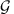, we classify the remaining nodes into the following three categories or states: (1) αt-nodes: nodes in FCCs; (2) βt-nodes: nodes that are APs in the GCC; (3) γt-nodes: nodes that are not APs in the GCC. Note that if a node is a γt-node, it must be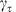-node with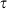<t. (The notations βt and γt here have totally different meanings from the critical exponents β and γ mentioned in the main text.)

According to the local tree approximation (Supplementary Note 4), the state of a randomly chosen node i can be determined by the states of its neighbours in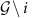, that is, the induced subgraph ofwith node i and all its links removed. In other words, in order to determine the state of a node, we need to know the states of its neighbours. Therefore, at each time step t, we need to know the probability that, following a randomly chosen link to one of its end nodes, this node belongs to any of the above categories after this link is removed. These probabilities are denoted as αt, βt, and γt, respectively. Note that for convenience sake here we use the same notation to denote both the state of a node and the probability of a node in that state. To be precise, hereafter when we consider the state of a neighbour of a given node i, we mean the state of the neighbour in the induced subgraph.

The GAPR process can be fully characterized by the three sets of probabilities {α0, α1,...}, {β0, β1,...} and {γ0, γ1,...}. Note that every node must belong to one of the three categories, which means the three sets of probabilities are not independent from each other. Specifically, at time step t, the probability γt can be derived by the other two sets of probabilities through the following normalization condition: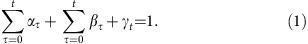Hereafter we focus on αt and βt only. We can calculate {α0, α1,...} and {β0, β1,...} in an iterative way. At first, we consider the initial time step t=0. The self-consistent equations for α0 and β0 are given by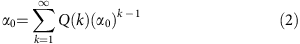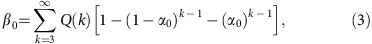where Q(k)=kP(k)/c is the degree distribution of the nodes that we arrive at by following a randomly chosen link (a.k.a. the excess degree distribution)1,2. We derive the above equations based on the following observations: (1) α0-node: its neighbours can only be α0-nodes; (2) β0-node: since it is an AP node, at least one of its neighbours is an α0-node. Moreover, since it belongs to the GCC, at lease one of its neighbours is not an α0-node.

For the t-th GAPR time step (t>0), we can compute αt and βt as follows: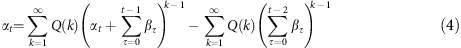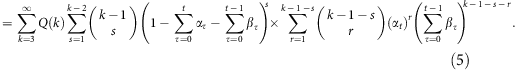The derivations of equations (4 and 5) are based on the following observations: (1) αt-node: First, its neighbours can only be αt-nodes or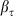-nodes with<t (because if one of its neighbours is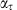-node with<t, this node will be an AP before time step t and hence would have already been removed; if one of its neighbour is βt-node, this node will belong to the GCC at time step t). Second, its neighbours can not be all-nodes with<t−1. Otherwise this node will be a leaf node before time step t−1, and will become an isolated node before the t-th time step. In this case, we can not reach this node through a randomly chosen link at time step t. (2) βt-node: First, its neighbours can not be-nodes with<t, otherwise this node would have been removed before time step t. Second, since it is an AP node, at least one of its neighbours is an αt-node. Finally, since this node belongs to the GCC, at least one of its neighbours is neither αt-node nor-node with<t.

Diagrammatic representations of these probabilities (αt, βt, γt) and their relationship are shown in Supplementary Figs 7–9 (see Supplementary Note 4 for details).

By solving the above self-consistent equations, we can obtain {α0, α1,...} and {β0, β1,...}, which govern the whole process of GAPR. With these two sets of probabilities, we can compute any quantities of interest, such as the total number of GAPR steps, the fraction of APs, the relative size of the GCC and the RGB, and so on (Supplementary Note 4; Supplementary Figs 10–12).

### Data availability

The data that support the findings of this study are available from the corresponding author on reasonable request.